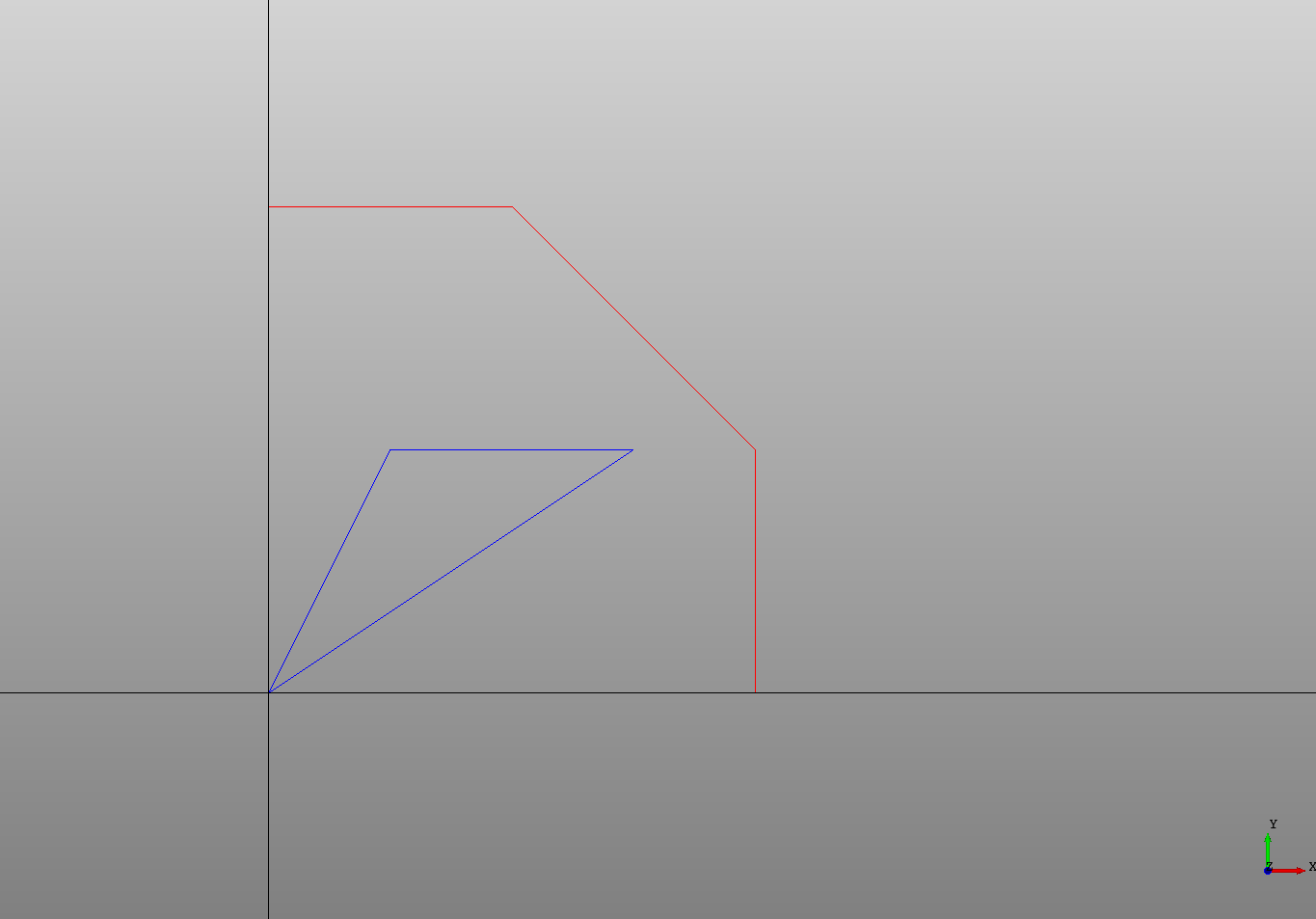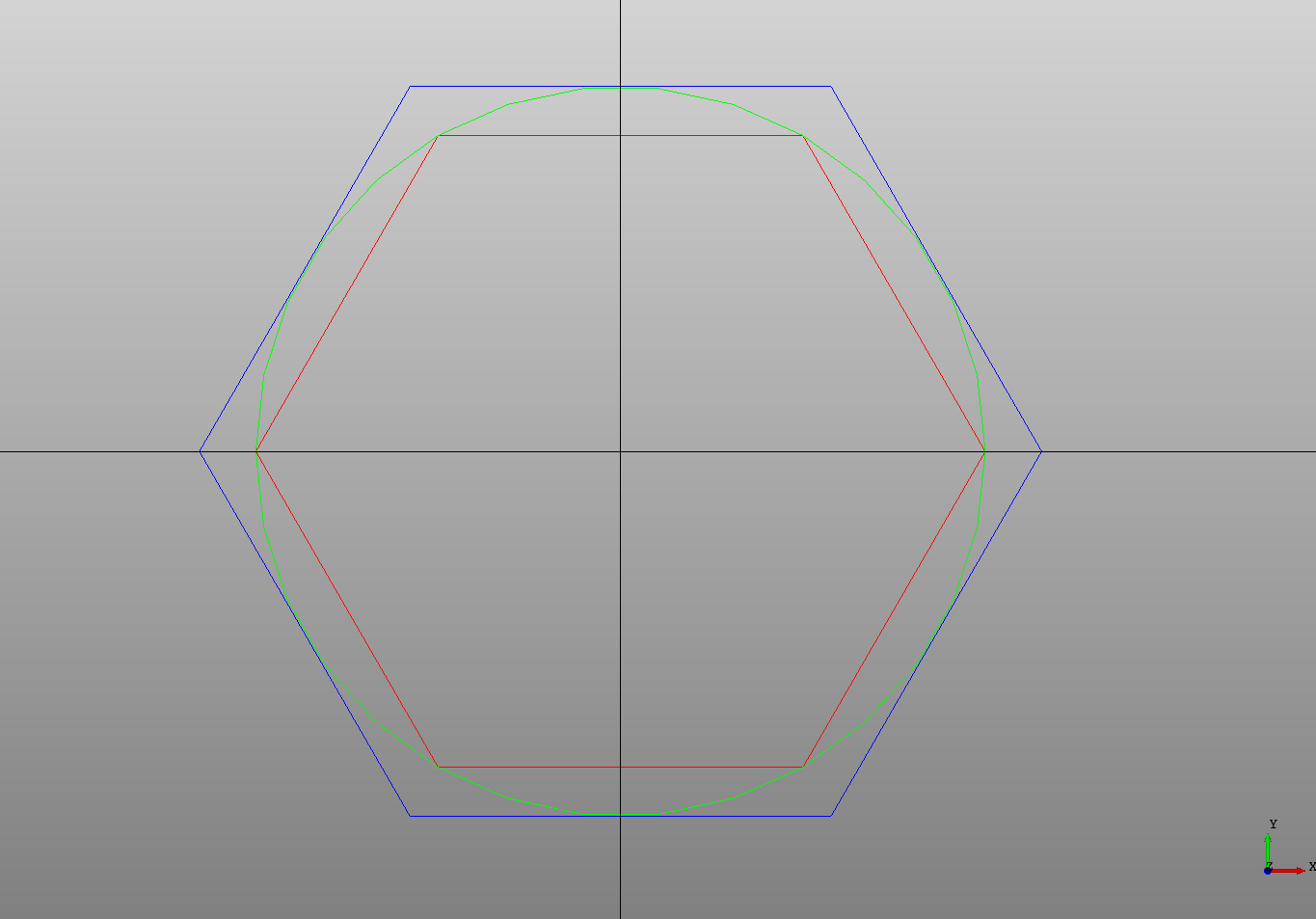## Polylines

A `Polyline` is a set of connected segments and is defined by a list of `points`. It may be set to `closed` to automatically set the last point to the first point.

``````# Created in DeclaraCAD
from math import pi

enamldef Assembly(Part):
Polyline:
color = 'red'
points = [(0, 0), (4, 0), (4, 2), (2, 4), (0, 4)]

Polyline:
color = 'blue'
points = [(0, 0), (1, 2), (3, 2)]
closed = True

# For reference
Line: xaxis:
color = 'black'
direction = (1, 0)
Line: yaxis:
color = 'black'
direction = (0, 1)
``````The points can be accessed like a normal python list which is useful for chaining multiple edges together into a wire.

## Polygons

DeclaraCAD also includes a `Polygon` which is just a closed `Polyline` with `count` points calculated for you that revolves around a `inscribed` or `circumscribed` `radius`.

This is best shown by example.

``````# Created in DeclaraCAD
from math import pi

enamldef Assembly(Part):
Circle: c1:
color = 'lime'
Polygon: p1:
color = 'red'
count = 6
Polygon: p2:
color = 'blue'
count = 6
inscribed = True

# For reference
Line: xaxis:
color = 'black'
direction = (1, 0)
Line: yaxis:
color = 'black'
direction = (0, 1)
``````This shows a circle and two polygons showing the difference between `circumscribed` (the default) and `inscribed`.